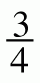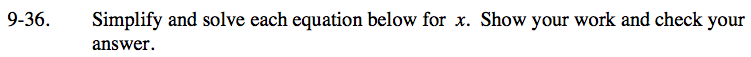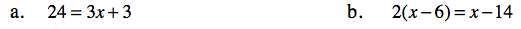Home > CC3 > Chapter 9 > Lesson 9.1.3 > Problem9-36

9-36.
1. Simplify and solve each equation below for x. Show your work and check your answer. Homework Help ✎

1. 24 = 3x + 3

2. 2(x − 6) = x − 14

3. 3(2x − 3) = 4x − 5

4.x = 2x − 5Begin isolating x by subtracting 3 from both sides.

24 = 3x + 3
-3 −3
21 = 3x

Divide both sides by 3.

$\frac{21}{3}=\frac{3{\it x}}{3}$

x = 7

Begin by distributing.

2(x) − 2(6) = x − 14
2x − 12 = x − 14

Add 12 to both sides.

2x = x − 2
Subtract x from each side.

x = −2Follow the methods presented in (a) and (b).

Follow the methods presented in (a) and (b).

x = 4